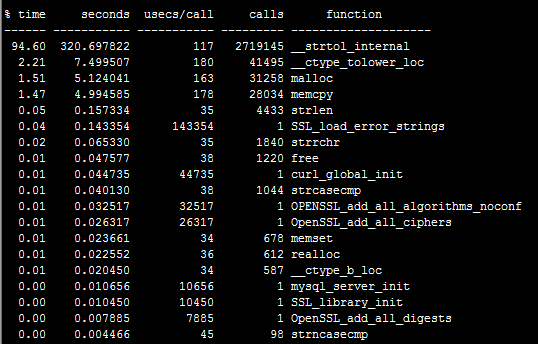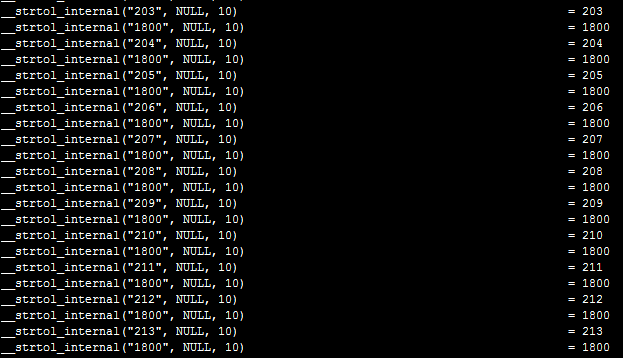# php in_array 的低性能PHP 首要说的就是性能方面的提升。对于 in_array()一直诟病很多，至于性能有多差，先简单测试一下，看看数据。

<?php/** * 获取当前时间戳（毫秒级） * @return float */function microtime_float(){    list($usec,$sec) = explode(' ', microtime());    return ((float)$usec + (float)$sec);}/** * 数组初始化 */$int_arr =$str_arr = [];for($i=0;$i<200000; $i++){$int_arr[] = $i;$str_arr[] = "{$i}";}$time_start = microtime_float();// 具体操作for($j=0;$j<3000; $j++){ if(in_array(18000,$int_arr)){        continue;    }//    if(isset($int_arr[$key])){//        continue;//    }}$time_end = microtime_float();echo '消耗时间：'.($time_end - $time_start);123456789101112131415161718192021222324252627282930 复制代码 测试结果 in_array 方式 1. 字符型字符串 // 3k 消耗时间：1.0687351226807 // 3w 消耗时间：10.569030046463 2. int 型字符串 // 3k 消耗时间：1.0500609874725 // 3w 消耗时间：10.290988922119 isset 方式 1. 字符型字符串 // 3k 消耗时间：0.00010299682617188 // 3w 消耗时间：0.00089907646179199 2. int 型字符串 // 3k 消耗时间：0.00010108947753906 // 3w 消耗时间：0.00085687637329102 结合上面测试数据，两种方式的性能差距还是挺明显的。 利用 ltrace 来跟踪进程调用库函数的情况： $ ltrace -c /usr/local/php/bin/php test.php看到库函数__strtol_internal 的调用非常之频繁，这个库函数__strtol_internal 是原来是 strtol 的别名，简单的说就是把字符串转换成长整形，可以推测 PHP 引擎已经检测到这是一个字符串型的数字，所以期望将他们转换成长整型来比较，这个转换过程中消耗了太多时间，我们再次执行:

$ltrace -e "__strtol_internal" /usr/local/php/bin/php test.php 复制代码 可以轻松抓到大量下图这样的调用，到此，问题找到了，in_array 这种松比较，会将两个字符型数字串先转换为长整型再进行比较，却不知性能就耗在这上面了。知道了症结所在，我们解决的办法就很多了，最简单的就是为 in_array 加第三个参数为 true，即变为严格比较，同时还要比较类型，这样避免了 PHP 自作聪明的转换类型，跑起来果然快多了，代码如下： in_array(search,array,type)参考上例，如改为：in_array(18000,$int_arr, true);in_array('18000', \$str_arr, true);分别执行3k次的消耗时间分别为：0.88052606582642、0.88974308967591234

总结一下，大数组的查询，用 in_array 函数是个糟糕的选择。应该尽量用 isset 函数来替代 。in_array 函数的复杂度是 O(n)，而 isset 函数的复杂度是 O(1)。## 评论